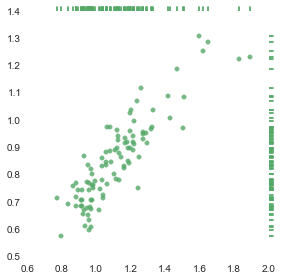Heinrich Hartmann

# A Categorical Perspective On Covariance

Written on 2014-12-08

For functions $f,g$ on a measure space $\Omega, \mu$ (e.g. a real interval $[0,1]$) there is a well known scalar product

This scalar product is of fundamental importance to the study of function and operators on measure spaces (Function Analysis). For example, there is a rich theory of how to decomposes functions on an interval into orthogonal Fourier-components.

If $\mu$ is a probability measure, we can regard $f,g$ as random variables. In this setting the viewpoint changes quite a bit. We give $f,g$ new names: $X,Y$. We are no longer interested in $\Omega$, and also rarely consider the scalar product. Instead the focus lies on expectation

and the covariance

This note considers the relation between these the probabilistic notions and the functional point of view.

## Constant Split

Let $\Omega, P$ be a probability space. Consider the vector space of square integrable functions:

See e.g. wikipedia for the details. We have the integral linear operator

The inclusion of the constant functions $\iota$ leaves us with a sequence:

whose composition $I \circ \iota = id_\IR$, since $P$ is a probability measure. We get an induced splitting of $\LL$ into constant functions plus functions with integral $I = 0$:

A function $x$ can be decomposed accordingly into its constant part $I(x)$ and its integral-0 part: $N(x) = x - I(x)$.

## Bilinear Forms

The space $\LL$ comes with a scalar product

which is non-degenerate and complete and thus makes this space into a Hilbert Space.

The covariance product is defined as

This product is bilinear but degenerate. The radical of $Cov$ are precisely the (almost) constant functions $\iota(\IR)$.

In the light of the above decomposition, we see that $Cov$ is the restriction of $S$ to $\LL_0$ extended back to $\LL$ using the projection $N(x) = x - I(x)$.

## Conclusion

• The space of integral-0 function $\LL_0$ together with the covariance product $Cov$ is a Hilbert Space.

• The natural inclusion $\LL_0 \rightarrow \LL$ is isometric with adjoint linear operator $N(x) = x - I(x)$.

• There is an orthogonal direct sum decomposition:

## Update 2015-05-17: Correlation as Cosine

The Pearson correlation is defined as:

It measures the linear dependece between two random variables. E.g. in the case of a discrete probability measure obtained from a sample, the correlation is the ratio between explained variance in a linear regression and total variance of the sample cf. wikipedia.Correlated (\rho ~ 0.88) and non-corrleated (\rho ~ -0.14) samples.

In analogy to the Euclidean plane, we define the cosine similarity between two functions by

Hence for centered functions $x,y \in \LL_0$ we have

which gives a surprising relation between two different geometric interpretations of the same data:

• Regrssion line of coefficient pairs $(x_i,y_i)$ in $\IR^2$
• Angle between vectors $(x_1,\dots,x_n)$ and $(y_1,\dots,y_n)$ in $\IR^n$.

Note, that for cetnered functions ($I(x) = I(y) = 0$) the regression line will always pass through the origin and the slope can be calculated to

However, at the moment I do not see how this helps to understand the above observation. My feeling is, that there should be a more conceptual reason for it. By interpreting $x,y$ as maps $\IR^2 \lra \IR^n$ we can bring duality theory for vector spaces into play and maybe gain more insight form this perspective.